# Prerequisites

In addition to the gravmagsubs package, this vignette uses the packages fields (Nychka et al. 2021), ggplot2 (Wickham 2016), gridExtra (Auguie 2017), scales (Wickham and Seidel 2022), and scatterplot3d (Ligges and Mächler 2003). Please make sure that fields, ggplot2, gridExtra, scales, and scatterplot3d are installed before following the vignette:

install.packages("fields")
install.packages("ggplot2")
install.packages("gridExtra")
install.packages("scales")
install.packages("scatterplot3d")

The gravmagsubs package must also be installed in order to follow the vignette. For instructions on installing gravmagsubs, see the README.

# Introducing gravmagsubs

This vignette briefly outlines the main functionality of the gravmagsubs package (Cronkite-Ratcliff, Phelps, and Scheirer 2022).

gravmagsubs is an R package for calculating the gravitational or magnetic anomaly at a predefined set of locations produced by a predefined set of rectangular prisms. The methods are:

• rectprismgrav(), a method that calculates the gravitational anomaly (the vertical component of the gravitational acceleration) at a location or series of locations given a prism or series of prisms, their input dimensions, and their density contrasts.
• rectprismmag(), a method that calculates the magnetic anomaly at a location or series of locations given a prism or series of prisms, their input dimensions, and their magnetization properties.

To import the gravmagsubs package into an R session:

library(gravmagsubs)

For help on how to use the package, use the following command to view the documentation for the package:

?gravmagsubs

or

help(gravmagsubs)

# rectprismgrav()

The rectprismgrav() function calculates the gravity anomaly from a series of 3-D rectangular prisms with vertical sides using the algorithm of Plouff (1975a). To view the arguments for rectprismgrav(), type the following:

args(rectprismgrav)

To see details about the function arguments, units, and output, access the help page by typing:

help(rectprismgrav)

or

?rectprismgrav

We begin by showing simple examples of calculating the gravity anomaly at a point, then along a line to generate 1-D anomalies from 2-D profiles of simple geologic models, then for more complex density models, before finally moving to 2-D anomalies of simple 3-D geologic models.

## rectprismgrav() for single calculations from a simple model

To calculate the gravity anomaly of simple models, it is only necessary to specify the measurement location (the “station”), the sides of the rectangular prism, and the density contrast.

Note that:

1. the edges of the prisms are always parallel to the standard Cartesian coordinate system,
2. spatial units are assumed to be km,
3. density units are assumed to be grams per cubic centimeter (g/cc), and
4. returned values are in milligals (mGal).

For example, we can calculate the gravity anomaly at a single station located at the origin.

# location of the point where the gravity anomaly will be calculated
gravstation <- data.frame(x=0, y=0, z=0)

We define a regular prism with dimensions of 10 km by 10 km in the horizontal dimensions and 5 km in the vertical dimension, whose top is at a depth of 5 km and is directly below the origin.

# the rectangular prism is defined by its six edges
prism1 <- data.frame(xmin=-5, xmax=5,
ymin=-5, ymax=5,
zmin=-10, zmax=-5)

We set the density contrast of the prism to be +0.3 g/cc,:

# density contrast in g/cc
drho <- 0.3

We then use the rectprismgrav() function to calculate the gravity anomaly at the station:

gravanom <- rectprismgrav(gravstation$x, gravstation$y, gravstation$z, prism1$xmin, prism1$xmax, prism1$ymin, prism1$ymax, prism1$zmin, prism1$zmax, drho) We can check the value of the gravity anomaly, in mGal, at the single station located at (0, 0, 0): gravanom ## [,1] ## [1,] 13.17689 To calculate the anomaly at another location, this time located away from the origin, we redefine the coordinates of the station and run rectprismgrav() again to obtain the new value. gravstation <- data.frame(x=-2, y=2, z=0) gravanom <- rectprismgrav(gravstation$x, gravstation$y, gravstation$z,
prism1$xmin, prism1$xmax,
prism1$ymin, prism1$ymax,
prism1$zmin, prism1$zmax, drho)

Since we are calculating the gravity anomaly further away from the center of mass of the prism, the gravity anomaly will be slightly weaker than before:

gravanom
##          [,1]
## [1,] 11.90794

## rectprismgrav() for simple cross-sectional models

Now consider the schematic of a vertical fault offset in a 2-D cross-section, centered at the origin, extending from -500 to +500 km in width and down to a depth of 4 km, with a fault offset of 1 km, an offset midpoint of 2 km depth, and a shallow-side depth of 1.5 km. The density contrast between the lower and upper unit is -0.67 g/cc. The formula for the gravity anomaly produced by a half slab in this situation (Lowrie 1997) is:

$(1) \qquad\qquad\qquad\qquad g = 2\rho\gamma h_1 \biggl(\frac{\pi}{2} + \tan^{-1}\biggl(\frac{x}{z_0}\biggr)\biggr)$

where $$g$$ is the gravitational anomaly in (mGal), $$\rho$$ is the density contrast (g/cc), $$h_1$$ is the thickness of the half slab (km), $$z_0$$ is the depth to the midpoint of the half-slab (km), $$x$$ is the location along the x-axis in kilometers, and $$\gamma$$ is the gravitational constant ($$\times$$ 1.e-11 m$$^3$$ kg$$^{-1}$$ s$$^{-2}$$) scaled to match the units of the other inputs.

The formula for the gravity anomaly produced by an infinite slab (Lowrie 1997) is:

$(2) \qquad\qquad\qquad\qquad g = 2\pi\rho\gamma h_2 \qquad\qquad\qquad\qquad$

where $$h_2$$ is the slab thickness (km).

Equation 2 can be added to Equation 1 to calculate the gravitational anomaly of the full cross-section:

$(3) \qquad\qquad\qquad\qquad g = 2\pi\rho\gamma h_2 + 2\rho\gamma h_1 \biggl(\frac{\pi}{2} + \tan^{-1}\biggl(\frac{x}{z_0}\biggr)\biggr)$

To calculate the gravity anomaly using Equation 3, define a data frame for the gravity station x-axis locations at which to measure the gravity anomaly:

grav.calc <- data.frame(X = seq(-25, by=.1, length=500)) 

The x-location of the gravity stations is given by grav.calc$X, and the y- and z-locations are both zero. Create the Y and Z coordinates of the gravity stations: grav.calc$Y <- 0
grav.calc$Z <- 0 Now calculate the anomaly according to Equation 3, where the half-slab thickness is the fault offset of 1 km, the midpoint to the half-slab is 2 km, the thickness of the infinite slab is 1.5 km, and the density contrast is -0.67 g/cc. rho <- -0.67 # density contrast (g/cc) gamma <- 6.674 # gravitational constant (x 1.e-11 m^3 / (kg s^2)) h1 <- 1.5 # infinite slab thickness (km) h2 <- 1 # fault offset (km) grav.calc$Geq <- 2*pi*rho*gamma*h1 + 2*rho*gamma*h2*(pi/2 + atan(grav.calc$X/2)) For the equivalent calculation using rectprismgrav(), create the identical cross-section, with prism center spacing at 1 km in the horizontal dimension and 100 m in the vertical dimension. prism.width.h <- 1 # horizontal prism width (km) prism.width.v <- 0.1 # vertical prism thickness (km) X1 <- seq(-499.5, 499.5, by=prism.width.h) Z1 <- seq(-3.95, -0.05, by=prism.width.v) XZr <- expand.grid(xcenter=X1, Y=0, zcenter=Z1) Now define the density contrast as -0.67 g/cc above the offset slab and 0 below it. To plot the section with the density contrast, we use the ggplot2 package (Wickham 2016) and the scales package (Wickham and Seidel 2022). XZr$density <- 0
XZr$density[XZr$zcenter > -h1] <- rho
XZr$density[XZr$zcenter > -(h1 + h2) & XZr$xcenter > 0] <- rho library(ggplot2) library(scales) # plot with custom color scale ggplot() + geom_raster(data = XZr, aes(x = xcenter, y = zcenter, fill = density)) + scale_fill_gradientn(colours=c("yellow", "darkred"), values = rescale(c(-0.67, -0.33, 0)), breaks = c(-0.67, 0), labels = paste(c(-0.67, 0)), limits = c(-0.67, 0), name = "") + geom_point(data = grav.calc, aes(x=X, y=Z)) + labs(x = "Distance [km]", y = "Depth [km]", title = "Density contrast (g/cc))") + theme(panel.background = element_rect(colour = "black", fill = "white"), panel.grid = element_blank(), plot.title = element_text(face = "bold", hjust = 0.5, size=16), aspect.ratio = 1/5)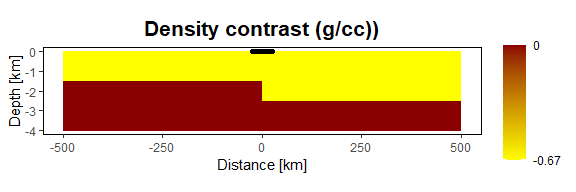This shows the density contrast of the resulting cross-section. The locations of the gravity stations are shown as black dots positioned above the fault offset. Calculate the gravity anomaly using rectprismgrav(). Extend the prisms +/- 500 km in the Y direction (perpendicular to the X-Z plane), to approximate a perfect 2-D model. grav.calc$Ggms <- as.vector(rectprismgrav(grav.calc$X, grav.calc$Y, grav.calc$Z, XZr$xcenter - prism.width.h/2,
XZr$xcenter + prism.width.h/2, XZr$Y - 500, XZr$Y + 500, XZr$zcenter - prism.width.v/2,
XZr$zcenter + prism.width.v/2, XZr$density) )

Compare the statistics of the two anomalies:

summary(grav.calc$Ggms) ## Min. 1st Qu. Median Mean 3rd Qu. Max. ## -69.41 -68.70 -55.86 -56.06 -43.45 -42.75 summary(grav.calc$Geq)
##    Min. 1st Qu.  Median    Mean 3rd Qu.    Max.
##  -69.52  -68.81  -55.97  -56.16  -43.56  -42.86

And the mean and standard deviation of their difference:

sd(grav.calc$Geq - grav.calc$Ggms)
##  0.0166237
mean(grav.calc$Geq - grav.calc$Ggms)
##  -0.1075556

We see that rectprismgrav() agrees quite well with the output of the theoretical equations for the idealized cross-section shown.

ggplot() +
geom_line(data = grav.calc, aes(x = X, y = Geq,
colour = "Calculated from equation")) +
geom_line(data = grav.calc, aes(x = X, y = Ggms,
colour = "Calculated from rectprismgrav()")) +
scale_colour_manual("", values=c("blue", "darkgreen")) +
labs(title = "", x = "Distance [km]", y = "Gravity anomaly (mGal)") +
theme(panel.background=element_rect(colour="black", fill="white"),
legend.key = element_rect(fill = "white"),
legend.position = c(0.2, 0.3),
panel.grid = element_blank(),
plot.title = element_text(face = "bold",hjust = 0.5, size=16 ),
aspect.ratio = 1/4)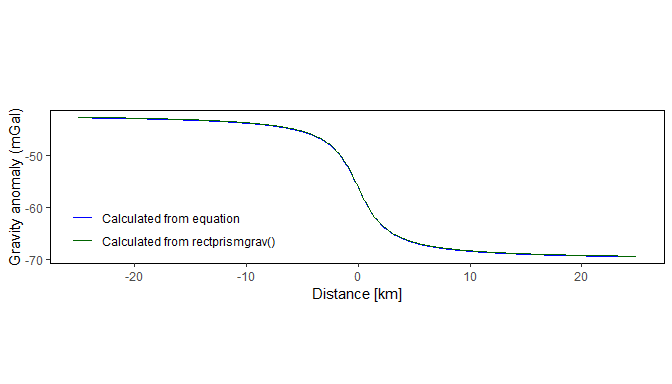Note that although the model extends to 500 km in either direction, the gravity stations are located within 25 km of the center of the model.

## rectprismgrav() for multiple complex cross-sectional models

### Using the sensitivity matrix to speed up calculations

An important feature of rectprismgrav() is the ability to calculate and save the sensitivity matrix. The calculation of the gravity anomaly can be factored into two matrices, the first of which, called the sensitivity matrix, represents the effect of the shape, orientation, and distance of the prisms from the measurement point (the gravity station). The second matrix represents the effect of the density contrast.

$(4) \qquad\qquad\qquad\qquad \bf{g} = \bf{G}\bf{\rho}$

where $$\bf{g}$$ is the gravity anomaly, $$\bf{\rho}$$ contains the density contrast of the individual prisms, and $$\bf{G}$$ is the sensitivity matrix. $$\bf{G}$$ has dimensions of M $$\times$$ N, where M is the number of gravity stations, and N is the number of prisms in the model. Matrix element i,j, called the sensitivity, represents the effect on the i-th gravity station by the j-th prism (Li and Oldenburg 1998). In this case, sensitivity has dimensions of acceleration per unit density. The vector $$\bf{\rho}$$ contains the density contrast of the j-th prism.

The calculation time is largely due to the time it takes to calculate the sensitivity matrix. By calculating the sensitivity matrix once, and saving the result, different density contrasts for the same prisms and stations can be explored rapidly, because only the matrix product needs to be calculated.

The rectprismgrav() function has a flag, bycell, which is FALSE by default. To obtain the sensitivity matrix, set bycell=TRUE and the density contrast to 1.

The ordering of the sensitivity matrix elements matches that of the inputs; the first row corresponds to the first gravity station, while the first column corresponds to the first prism, the second column to the second prism, etc.

Using the previous example, add a second column of density values to XZr.

XZr$density1 <- 1 Now calculate the sensitivity matrix, using the new density values and the bycell=TRUE option. XZr.sensmat <- rectprismgrav(grav.calc$X, grav.calc$Y, grav.calc$Z,
XZr$xcenter - prism.width.h/2, XZr$xcenter + prism.width.h/2,
XZr$Y - 500, XZr$Y + 500,
XZr$zcenter - prism.width.v/2, XZr$zcenter + prism.width.v/2, XZr$density1, bycell=TRUE) dim(XZr.sensmat) ##  500 40000 To visualize the sensitivity matrix for the tenth gravity station, for example, we can plot the cross-section and use the sensitivity values to color the prisms. (Because the values are highly skewed, we assign the color scale according to the log of the sensitivity, so that the highest values are in red). # create a data frame with locations and log of the 10th gravity station xzs1 <- data.frame(x=XZr[,1], z=XZr[,3], sensiv=log10(XZr.sensmat[10,])) # create the plot ggplot() + geom_raster(data = as.data.frame(xzs1) , aes(x = x, y = z, fill = sensiv)) + scale_fill_gradientn(colours=c("yellow","orange","red", "darkred"), name="") + geom_point(data = grav.calc, aes(x=X, y=Z), colour="darkgray") + geom_point(data = grav.calc[10,], aes(x=X, y=Z), colour="black") + labs(x = "Distance [km]", y = "Depth [km]", title = "log(Sensitivity(mGal per g/cc))") + theme(panel.background=element_rect(colour="black", fill="white"), panel.grid = element_blank(), plot.title = element_text(face = "bold",hjust = 0.5, size=16 ), aspect.ratio=1/4)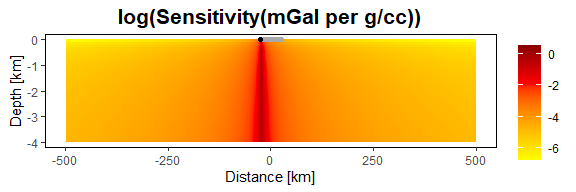The locations of the gravity stations are shown as gray dots positioned above the center of the model, with the location of the tenth station shown in black. The effect of the prisms is related to their position relative to the gravity station, with prisms directly below the station contributing the most to the z-component of the gravitational acceleration. Now that the sensitivity matrix is stored, experimenting with multiple densities is a matrix multiplication operation. For example, suppose the average slab density in the previous model is uncertain, and may range from a low value of -0.9 g/cc to a high value of -0.4 g/cc. We can add these end-member values to the data frame. XZr$density2 <- 0

We create new columns in the data frame to represent these alternate density values. Then we assign slab values to -0.9 g/cc and -0.4 g/cc in the new columns to represent these new models:

XZr$density2[XZr$density == rho] <- -0.9
XZr$density3 <- 0 XZr$density3[XZr$density == rho] <- -0.4 There are now three models of average slab densities for the cross-section: -0.67 g/cc, -0.9 g/cc, and -0.4 g/cc, located in the three density columns of the XZr data frame. Multiplying these models by the sensitivity matrix will yield and M $$\times$$ P matrix of M gravity stations and P models (note the data frame must be converted to a matrix before performing the calculations). gravanom.models <- XZr.sensmat %*% as.matrix(XZr[,c("density", "density2", "density3")]) Plot the results. grav.calc$gmod.orig <- gravanom.models[,1]
grav.calc$gmod.low <- gravanom.models[,2] grav.calc$gmod.high <- gravanom.models[,3]

ggplot() +
geom_line(data = grav.calc, linewidth=2,
aes(x = X, y = gmod.low, colour = "-0.9 g/cc")) +
geom_line(data = grav.calc, linewidth=2,
aes(x = X, y = gmod.orig, colour = "-0.67 g/cc")) +
geom_line(data = grav.calc, linewidth=2,
aes(x = X, y = gmod.high, colour = "-0.4 g/cc")) +
scale_colour_manual(name="Density contrast", values=c("blue", "black", "red")) +
labs(title = "", x = "Distance [km]", y = "Gravity anomaly (mGal)") +
theme(panel.background=element_rect(colour="black", fill="white"),
legend.key = element_rect(fill = "white"),
legend.position = "right",
panel.grid = element_blank(),
plot.title = element_text(face = "bold", hjust = 0.5, size=16 ),
aspect.ratio = 1/4)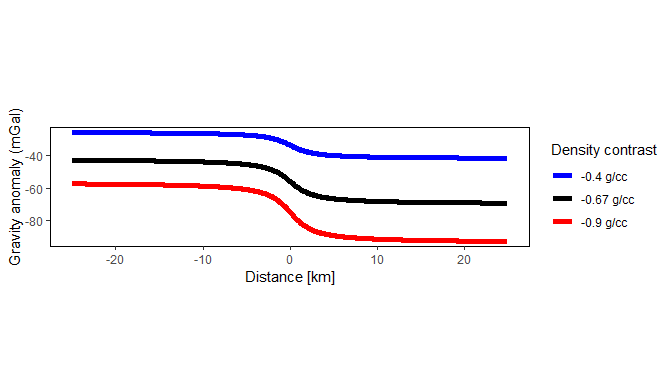### Generating multiple gravity anomalies for complex cross-sectional models

It may be of interest to calculate the gravity anomaly of more complex geologic models. Suppose, for example, a sedimentary basin is filled with a mix of variably dense lenses of alluvial fan deposits. There is not enough information to map these deposits in the subsurface, but their distribution could be simulated with a Gaussian random field. The package fields (Nychka et al. 2021) can be used to generate these models.

library(fields)
set.seed(123)

For this example the Gaussian random field is defined as having a correlation length of 300 m in the vertical direction and 15 km in the horizontal direction. The realizations will have Gaussian distributions for density that are very similar to each other, but they will differ in the spatial distribution of the values. Similar values will tend to cluster according to the parameters of the Gaussian random field model. In this case, the clusters will tend towards dimensions of 15 km horizontal by 300 m vertical.

Specify the parameters of the random field model.

# setting the anisotropy matrix
aniso.mat <- matrix(c(15/prism.width.h, 0, 0, 0.3/prism.width.v), nrow=2, byrow=TRUE)
# need to re-format the simulation grid using indices
rf.grid <- list(x = 1:length(X1), y = 1:length(Z1))
# setting up the random field model
rf.model <- stationary.image.cov(setup=TRUE, grid = rf.grid, V=aniso.mat )

Generate two realizations of subsurface density using this random field model, for the same set of prisms used in the previous section. By default, the random field will have a mean of zero and a standard deviation of approximately 1 g/cc. We can re-scale the results to achieve a desired standard deviation of density, in this case a more realistic standard deviation of 0.14 g/cc (variance of 0.02 g/cc).

std.dev <- 0.14
grav.sim.1 <- sim.rf(rf.model) * std.dev
grav.sim.2 <- sim.rf(rf.model) * std.dev

The mean density of each realization is approximately zero, and the standard deviation is approximately 0.14 g/cc.

Plot the results:

library(gridExtra)

# Create the plot with the title: sim1 density contrast (g/cc)

xzg <- data.frame(x=XZr[,1], z=XZr[,3],
gravsim1=c(grav.sim.1),
gravsim2=c(grav.sim.2))

g1.plot<- ggplot() +
geom_raster(data = as.data.frame(xzg), aes(x = x, y = z, fill = gravsim1)) +
breaks = c(-0.5, 0, 0.5),
labels = paste(c(-0.5, 0 ,0.5)),
name = "") +
geom_point(data = grav.calc, aes(x=X, y=Z)) +
labs(x = "Distance [km]",
y = "Depth [km]",
title = "sim1 density contrast (g/cc)") +
theme(panel.background=element_rect(colour="black", fill="white"),
panel.grid = element_blank(),
plot.title = element_text(face = "bold", hjust = 0.5, size=16 ),
legend.position = "bottom",
aspect.ratio = 1/5)

# Create the plot with the title: sim2 density contrast (g/cc)

g2.plot<- ggplot() +
geom_raster(data = as.data.frame(xzg), aes(x = x, y = z, fill = gravsim2)) +
breaks = c(-0.5, 0, 0.5),
labels = paste(c(-0.5, 0, 0.5)),
name = "") +
geom_point(data = grav.calc, aes(x=X, y=Z)) +
labs(x = "Distance [km]",
y = "Depth [km]",
title = "sim2 density contrast (g/cc)") +
theme(panel.background = element_rect(colour="black", fill="white"),
panel.grid = element_blank(),
plot.title = element_text(face = "bold", hjust = 0.5, size=16 ),
legend.position = "bottom",
aspect.ratio = 1/5)

grid.arrange(g1.plot, g2.plot, nrow=2)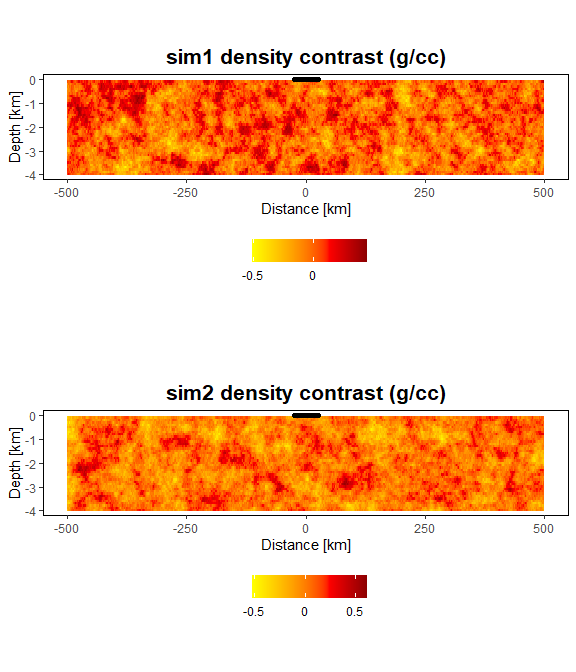The locations of the gravity stations are shown as black dots positioned above the center of the model.

Taking advantage of the sensitivity matrix, the gravity anomalies for the two realizations can now be calculated by matrix multiplication. First, however, we can stack the two realizations into a single matrix so that the gravity anomaly can be computed once on all realizations.

grav.sims.mat <- matrix(c(grav.sim.1, grav.sim.2) , byrow=FALSE, ncol =2)
grav.sims.anom <- XZr.sensmat %*% grav.sims.mat
dim(grav.sims.anom)
##  500   2

The dimensions of the output represent 500 gravity stations and 2 resulting gravity anomaly profiles.

Now we plot the anomalies from the two realizations. From the plots, it can be seen that a 4 km thickness of sediments, with the density distributions specified, produce gravity anomalies that exhibit quasi-periodic behavior, with an amplitude of up to approximately 10 mGal. The quasi-periodic behavior is the result of the spatial aggregation of like density values within the sediments.

anom.df <- data.frame(x = grav.calc$X, anom1 = grav.sims.anom[,1], anom2 = grav.sims.anom[,2]) g1.plot<- ggplot() + geom_line(data = as.data.frame(anom.df), aes(x = x, y = anom1)) + labs(x = "Distance [km]", y = "Anomaly (mGal)", title = "sim1 anomaly profile (mGal)") + theme(panel.background = element_rect(colour="black", fill="white"), panel.grid = element_blank(), plot.title = element_text(face = "bold", hjust = 0.5, size=16 ), legend.position = "bottom", aspect.ratio = 1/5) g2.plot<- ggplot() + geom_line(data = as.data.frame(anom.df), aes(x = x, y = anom2)) + labs(x = "Distance [km]", y = "Anomaly (mGal)", title = "sim2 anomaly profile (mGal)") + theme(panel.background = element_rect(colour="black", fill="white"), panel.grid = element_blank(), plot.title = element_text(face = "bold", hjust = 0.5, size=16 ), legend.position = "bottom", aspect.ratio = 1/5) grid.arrange(g1.plot, g2.plot, nrow=2)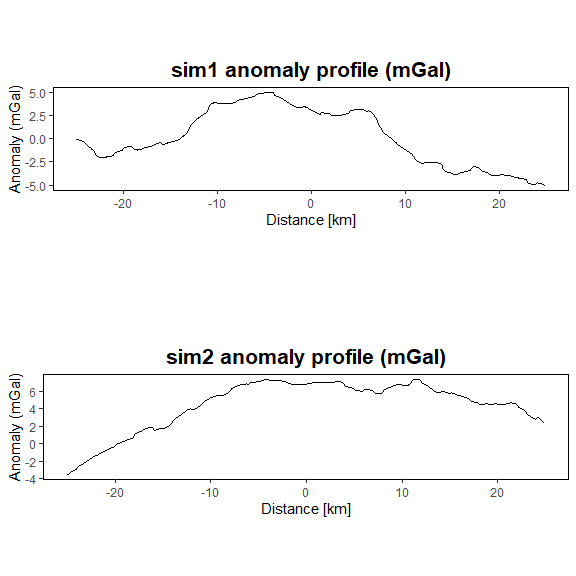## rectprismgrav() for 3-D models The generation of 2-D gravitational anomalies from 3-D source bodies parallels that of the generation of 1-D anomalies from 2-D source bodies. First, generate a 2-D grid of gravity stations, with elevation of zero. xstations <- seq(-5, 5, by=0.1) ystations <- seq(-5, 5, by=0.1) D3.stations <- expand.grid(xstations, ystations) D3.stations$Zstation <- 0
names(D3.stations) <- c("Xstation", "Ystation", "Zstation")

Then create the source body, in this case a 3-D rectangular body 4 km in the X and Y dimensions and 2 km in the Z dimension, centered at the origin and with a top located 1 km below the surface, with the density contrast ranging from -0.2 g/cc to 0.2 g/cc and increasing along the y-axis. The prisms each measure 0.1 km on a side. Plot the prism centers using scatterplot3d(), from the scatterplot3d package (Ligges and Mächler 2003).

width <- 0.1
half.width <- width / 2

xsource <- seq(-2 + half.width, 2 - half.width, by=width)
ysource <- seq(-2 + half.width, 2 - half.width, by=width)
zsource <- seq(-3 + half.width, -1 - half.width, by=width)

D3.source <- expand.grid(xsource, ysource, zsource)
names(D3.source) <- c("xcenter", "ycenter", "zcenter")

D3.source$density <- 0.1 * D3.source$ycenter

# define the color ramp by splitting the density model into 10 equally-spaced intervals
yorPal <- colorRampPalette(c('khaki', 'orange', 'firebrick'))
D3.cols = cut(D3.source$density, breaks=10) img3d <- scatterplot3d::scatterplot3d(D3.source$xcenter,
D3.source$ycenter, D3.source$zcenter,
pch=16, cex.symbols=.5,
color=yorPal(10)[as.numeric(D3.cols)],
xlab="Distance [km]", ylab="[km]", zlab="[km]")

leg.minmax <- c(paste("+", max(D3.source$density), " g/cc", sep=""), " 0.0 g/cc", paste(min(D3.source$density), "g/cc"))
legend(img3d$xyz.convert(3.7, -2, -2.7), legend = leg.minmax, col=c("firebrick", "orange", "khaki"), pch=16, title="Density contrast", inset = -0.25, xpd = TRUE, bty="n")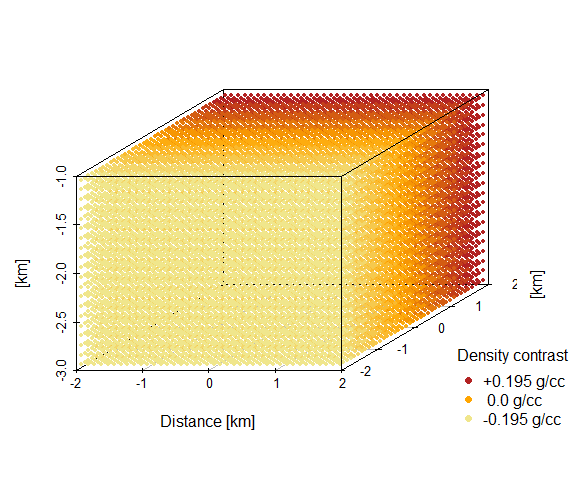Calculate the gravity anomaly associated with the prism and plot it. Note that we defined the prisms based on their center coordinates, but we need to provide the coordinates of the cell edges (xmin, xmax, ymin, ymax, zmin, zmax). We find the edges by adding or subtracting half of the prism width to the prism centers. D3.gravanom <- rectprismgrav(D3.stations$Xstation,
D3.stations$Ystation, D3.stations$Zstation,
D3.source$xcenter - half.width, D3.source$xcenter + half.width,
D3.source$ycenter - half.width, D3.source$ycenter + half.width,
D3.source$zcenter - half.width, D3.source$zcenter + half.width,
D3.source$density, bycell=FALSE) # create data frame d3.st <- data.frame(x=D3.stations$Xstation, y=D3.stations$Ystation, val1 = D3.gravanom) # plot with custom color scale ggplot() + geom_raster(data = d3.st, aes(x = x, y = y, fill = val1)) + scale_fill_gradientn( colours = c("darkblue", "blue", "skyblue", "white", "tomato", "red", "darkred"), values = rescale(c(-1.5, 0, 1.5)), breaks = seq(-1.5, 1.5, 0.5), labels = paste(seq(-1.5, 1.5, 0.5)), limits = c(-1.6, 1.6), name = "") + labs(x = "Easting [km]", y = "Northing [km]", title = "Gravity anomaly (mGal)") + theme(panel.background = element_rect(colour = "black", fill = "white"), panel.grid = element_blank(), plot.title = element_text(face = "bold", hjust = 0.5, size=16), aspect.ratio = 1)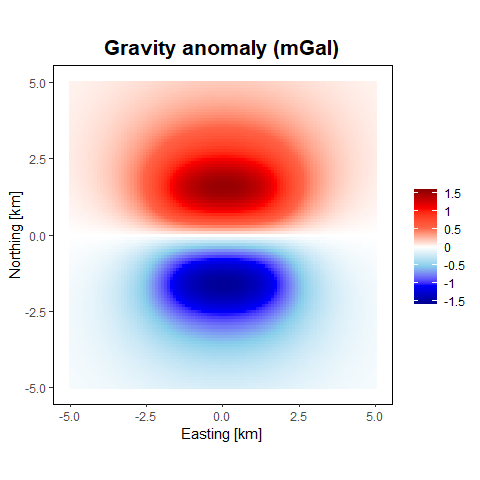# rectprismmag() The rectprismmag() function calculates the magnetic field anomaly from a series of 3-D rectangular prisms with vertical sides using the algorithm of Plouff (1975b). Calculating the magnetic field anomaly follows the same approach as that for gravity, except that additional parameters need to be specified for the magnetization properties instead of the density contrast. To view the arguments for rectprismmag(), type the following: args(rectprismmag) To see details about the function arguments, units, and output, access the help page by typing: help(rectprismmag) or ?rectprismmag ## rectprismmag() for a single induced source body To calculate the magnetic anomaly, in addition to the station locations and source body edges, the remanent magnetization at each prism needs to be specified along with the properties of the inducing field. We use the same dimensions as the 3-D gravity model from the previous section, in this case assuming that the model has a normally distributed, spatially uncorrelated, magnetic susceptibility with an average of 0.015 SI units, and a standard deviation of 0.001 SI units. The ambient field is given a constant intensity of 48,800 nT, with inclination of 60 degrees (measured from horizontal downwards) and declination of 12 degrees (positive clockwise from true north). Add the susceptibility values to the data frame defining the prisms. D3.source$suscnorm <- rnorm(n=length(D3.source[,1]), mean=0.015, sd=0.001)

Run rectprismmag() and plot the results. Note that all values for the magnetic field (suscvolsi, nrmstr, nrmdecl, nrmincl, fieldtotal, fielddecl, fieldincl) are vectors which must have one entry per prism. Here, because the values are constant, we supply them directly to the data frame.

D3.source$nrmstr <- 0 D3.source$nrmdecl <- 0
D3.source$nrmincl <- 0 D3.source$fieldtotal <- 48800
D3.source$fielddecl <- 12 D3.source$fieldincl <- 60

D3.maganomind <- rectprismmag(D3.stations$Xstation, D3.stations$Ystation,
D3.stations$Zstation, D3.source$xcenter - half.width,
D3.source$xcenter + half.width, D3.source$ycenter - half.width,
D3.source$ycenter + half.width, D3.source$zcenter - half.width,
D3.source$zcenter + half.width, suscvolsi = D3.source$suscnorm,
nrmstr = D3.source$nrmstr, nrmdecl = D3.source$nrmdecl,
nrmincl = D3.source$nrmincl, fieldtotal = D3.source$fieldtotal,
fielddecl = D3.source$fielddecl, fieldincl = D3.source$fieldincl,
bycell=FALSE)

# create data frame
d3.st <- D3.stations
d3.st$val <- D3.maganomind # plot with custom color scale ggplot() + geom_raster(data = as.data.frame(d3.st), aes(x = Xstation, y = Ystation, fill = val)) + scale_fill_gradientn( colours = c("darkblue", "blue", "lightblue", "white", "pink", "red", "darkred"), values = rescale(c(min(d3.st$val), 0, max(d3.st$val))), breaks = c(-50, 0, 50, 100), labels = paste(c("-50", 0, "50", "100")), limits = c(-50, max(d3.st$val)),
name = "") +
labs(x = "Distance [km]",
y = "Distance [km]",
title = "Induced magnetic anomaly (nT)") +
theme(panel.background = element_rect(colour="black", fill="white"),
panel.grid = element_blank(),
plot.title = element_text(face = "bold", hjust = 0.5, size=16),
aspect.ratio = 1)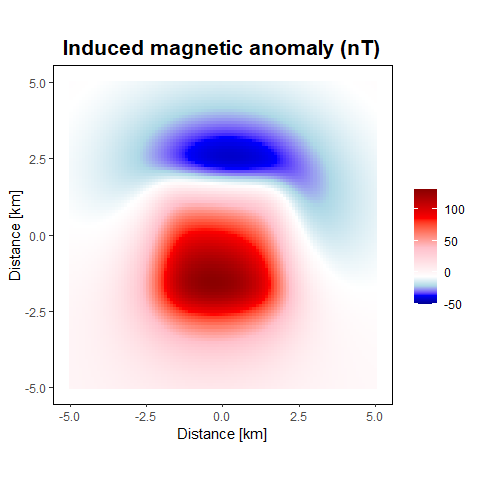## rectprismmag() for a single remanently magnetized source body with an induced component

Next we reproduce the example shown in Fig. 1 of Gonzalez, Barbosa, and Oliveira (2022), which consists of a prism with both induced and remanent magnetization. The prism measures 2 km in the X dimension, 2.5 km in the Y dimension, and 1.95 km in the Z dimension, with the top of the prism located 0.55 km below the surface. The ambient field has an intensity of 23,639 nT, an inclination of -45 degrees, and a declination of -30 degrees. The prism has a magnetic susceptibility of 0.1 (SI units). The prism also has a remanent magnetization of 5 A/m, and the remanent magnetic field has an inclination of -30 degrees and a declination of -15 degrees. We do not add Gaussian noise to our example as done by Gonzalez, Barbosa, and Oliveira (2022).

xstations <- seq(-5, 10, by=0.25)
ystations <- seq(-6, 10, by=0.25)
stations <- expand.grid(xstations, ystations)
stations$Zstation <- 0 names(stations) <- c("Xstation", "Ystation", "Zstation") maganomnrm <- rectprismmag(stations$Xstation,
stations$Ystation, stations$Zstation,
xmin = 1,
xmax = 3,
ymin = 1,
ymax = 3.5,
zdeep = -2.5,
zshallow = -0.55,
suscvolsi = 0.1,
nrmstr = 5,
nrmdecl = -15,
nrmincl = -30,
fieldtotal = 23639,
fielddecl = -30,
fieldincl = -45,
bycell=FALSE)

# create data frame
d3.st <- data.frame(x=stations[,1], y=stations[,2], val1 = maganomnrm)

# plot with custom color scale
ggplot() +
geom_raster(data = d3.st, aes(x = x, y = y, fill = val1)) +
colours = c("darkblue", "blue", "skyblue", "white", "tomato", "red", "darkred"),
values = rescale(c(-1000, 0,1500)),
breaks = c(-1000, -500, 0, 500, 1000, 1500),
labels = paste(c(-1000, -500, 0, 500, 1000, 1500)),
limits = c(-1000, 1500),
name = "") +
labs(x = "Easting [km]",
y = "Northing [km]",
title = "Total magnetic anomaly (nT)") +
theme(panel.background = element_rect(colour = "black", fill = "white"),
panel.grid = element_blank(),
plot.title = element_text(face = "bold", hjust = 0.5, size=16),
aspect.ratio = 1)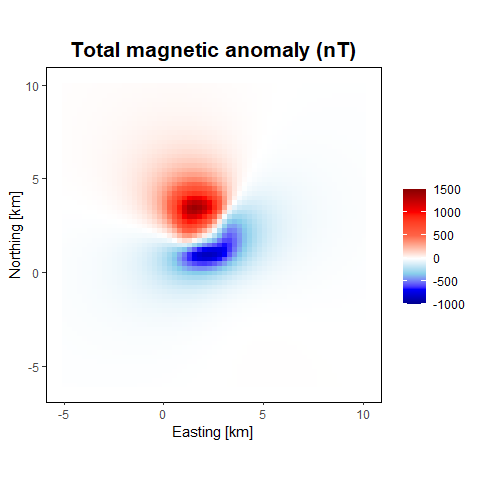# Summary

The purpose of this vignette has been to show how to apply the gravmagsubs functions to calculate gravitational and magnetic anomalies. Methods of calculating anomalies at discrete points, and across arrays of regularly spaced points, have been demonstrated.

In general the calculation time is rapid (a few seconds) for models with tens of thousands of rectangular prisms and tens of thousands of station locations, when calculated on a scientific laptop (64-bit Intel I9 processor (2.40 GHz) with 64 GB RAM). For gravity, calculation time can be improved by taking advantage of the sensitivity matrix, which allows for rapid calculations of multiple density models given the same set of prisms.

# References

Auguie, B. 2017. gridExtra: Miscellaneous Functions for "Grid" Graphics. https://CRAN.R-project.org/package=gridExtra.
Cronkite-Ratcliff, C., G. Phelps, and D. Scheirer. 2022. Gravmagsubs: Gravitational and Magnetic Attraction of 3-d Vertical Rectangular Prisms (version 1.0). Reston, VA: U.S. Geological Survey; U.S. Geological Survey. https://doi.org/10.5066/P9HBSPRM.
Gonzalez, S. P., V. C. F. Barbosa, and V. C. Oliveira. 2022. “Analyzing the Ambiguity of the Remanent-Magnetization Direction Separated into Induced and Remanent Magnetic Sources.” Journal of Geophysical Research: Solid Earth 127 (e2022JB024151). https://doi.org/10.1029/2022JB024151.
Li, Y., and D. Oldenburg. 1998. 3-D Inversion of Gravity Data.” Geophysics 63: 109–19. https://doi.org/10.1190/1.1444302.
Ligges, U., and M. Mächler. 2003. scatterplot3d - an R Package for Visualizing Multivariate Data.” Journal of Statistical Software 8 (11): 1–20. https://doi.org/10.18637/jss.v008.i11.
Lowrie, W. 1997. Fundamentals of Geophysics. Cambridge University Press.
Nychka, D., R. Furrer, J. Paige, and S. Sain. 2021. fields: Tools for Spatial Data.” Boulder, CO, USA: University Corporation for Atmospheric Research. https://github.com/dnychka/fieldsRPackage.
Plouff, D. 1975a. “Derivation of Formulas and FORTRAN Programs to Compute Gravity Anomalies of Prisms.” No. PB-243-526. National Technical Information Service. https://ntrl.ntis.gov/NTRL/dashboard/searchResults/titleDetail/PB243526.xhtml.
———. 1975b. “Derivation of Formulas and FORTRAN Programs to Compute Magnetic Anomalies of Prisms.” No. PB-243-525. National Technical Information Service. https://ntrl.ntis.gov/NTRL/dashboard/searchResults/titleDetail/PB243525.xhtml.
Wickham, H. 2016. ggplot2: Elegant Graphics for Data Analysis. Springer-Verlag New York. https://ggplot2.tidyverse.org.
Wickham, H., and D. Seidel. 2022. scales: Scale Functions for Visualization. https://scales.r-lib.org.